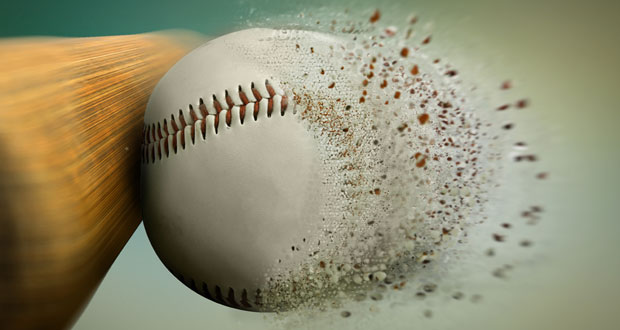# Impulse & Force Time Graphs In SportImpulse is simply a measure of the force applied for a specific time. Impulse = force x time and has units Ns (Newton seconds). It is an important concept in sport because many techniques, particularly throwing activities, require the performer to apply as large a force possible for as long as possible.

The area of a force-time graph gives the impulse. For example, when hitting a golf ball the player needs to strike the ball as hard as possible (applying a large force) as the ball will fly off in a very short time. The golfer cannot really control how long the force is applied to the ball but they can increase the magnitude of the force.

However, a Discus thrower needs to apply a force to the discus for as long as possible to put the most energy into the implement. The same is true for the Javelin thrower. The longer ‘pull’ they can apply to the object the faster it will leave the hand.

### Increasing the Impulse

Let’s look at the example of a cricketer throwing a ball. There are two ways they can increase the impulse. One is applying a greater force (e.g. moving the arm faster) which is likely to involve becoming stronger. The other way is to adjust the technique so the force is applied over a longer time. So for our cricketer, beginning the throw with the arm fully back and releasing as far forwards with a final flick of the fingers). However, be careful, there is little point increasing the time the force is applied to the ball if the arm is moving slower (i.e. the applied force is decreased).

#### Example Calculations

The cricketer applies a force of 20N to a ball for 0.5 seconds. What is the impulse?

Impulse = force x time

I = 20 x 0.5 = 10Ns

A hammer thrower applies a force of 30N for 1 second doing 1 turn and 30N for 3 seconds doing 4 turns. What is the difference in impulse?

I (1 turn) = 30 x 1 = 30 Ns

I (4 turns) = 30 x 3 = 90 Ns

So, we can see that three times more impulse is generated by doing four turns as opposed to one turn. The force is applied to the hammer for longer increasing impulse.

• Use your knowledge of impulses to explain whether a tennis ball struck with a backhand without following through with the racket will hit the ball harder than a backhand, where the player follows the shot through?

## Force time graphs

Force time graphs are often used to illustrate impulse. The area of the graph = impulse.

The graph below shows the force-time plot for a vertical jump. When the athlete is standing still only weight from gravity is acting down. As they bend their legs ready to jump the force on the ground is negative and then when they explode upwards into the jump the force becomes positive again.